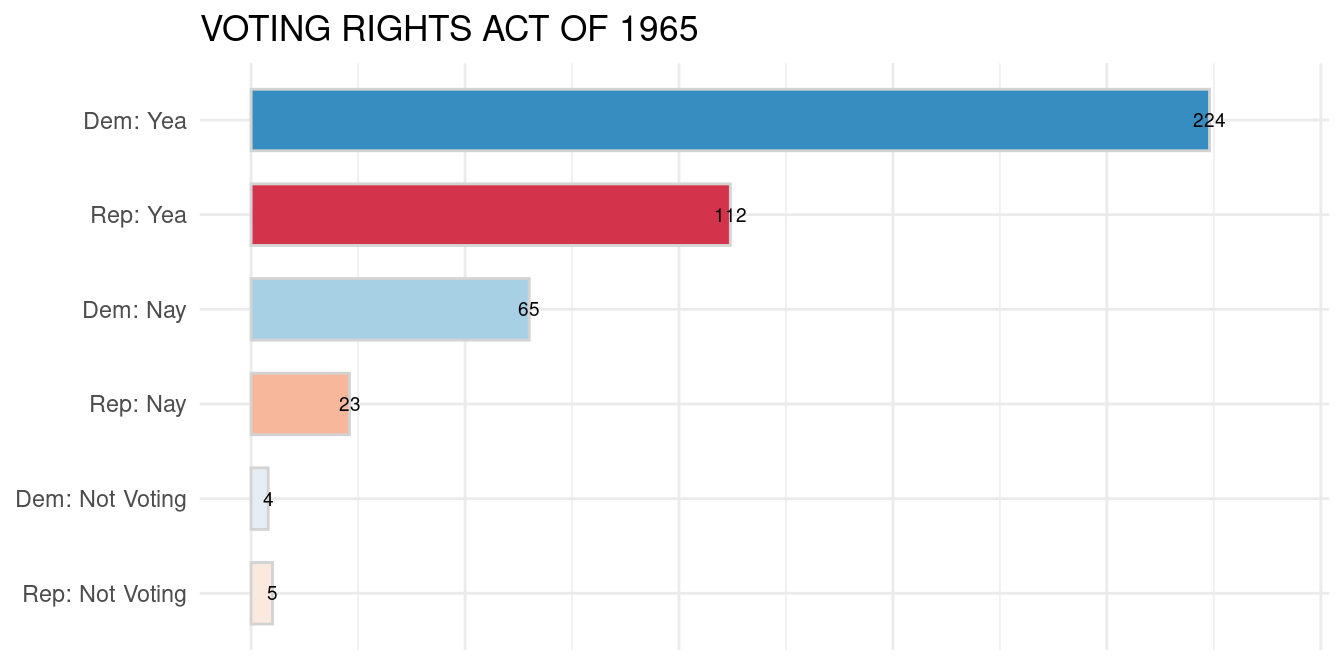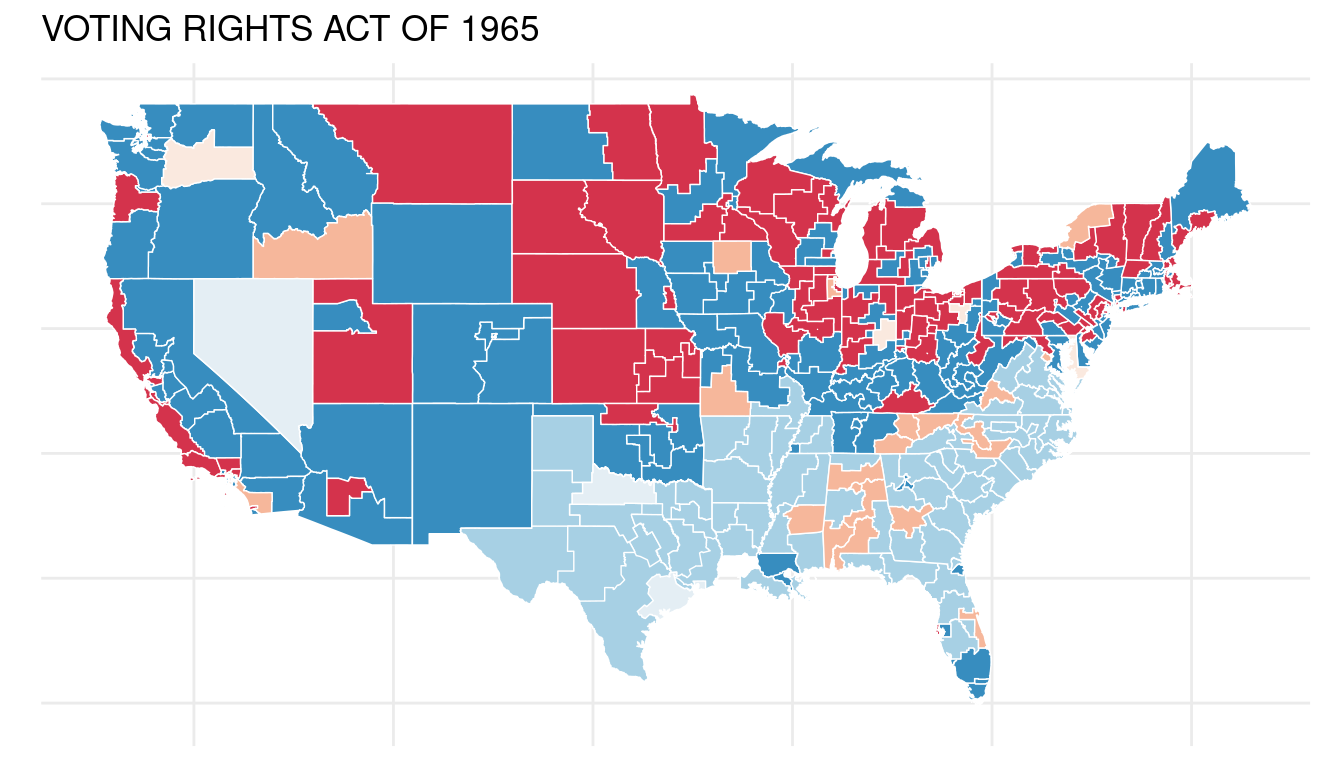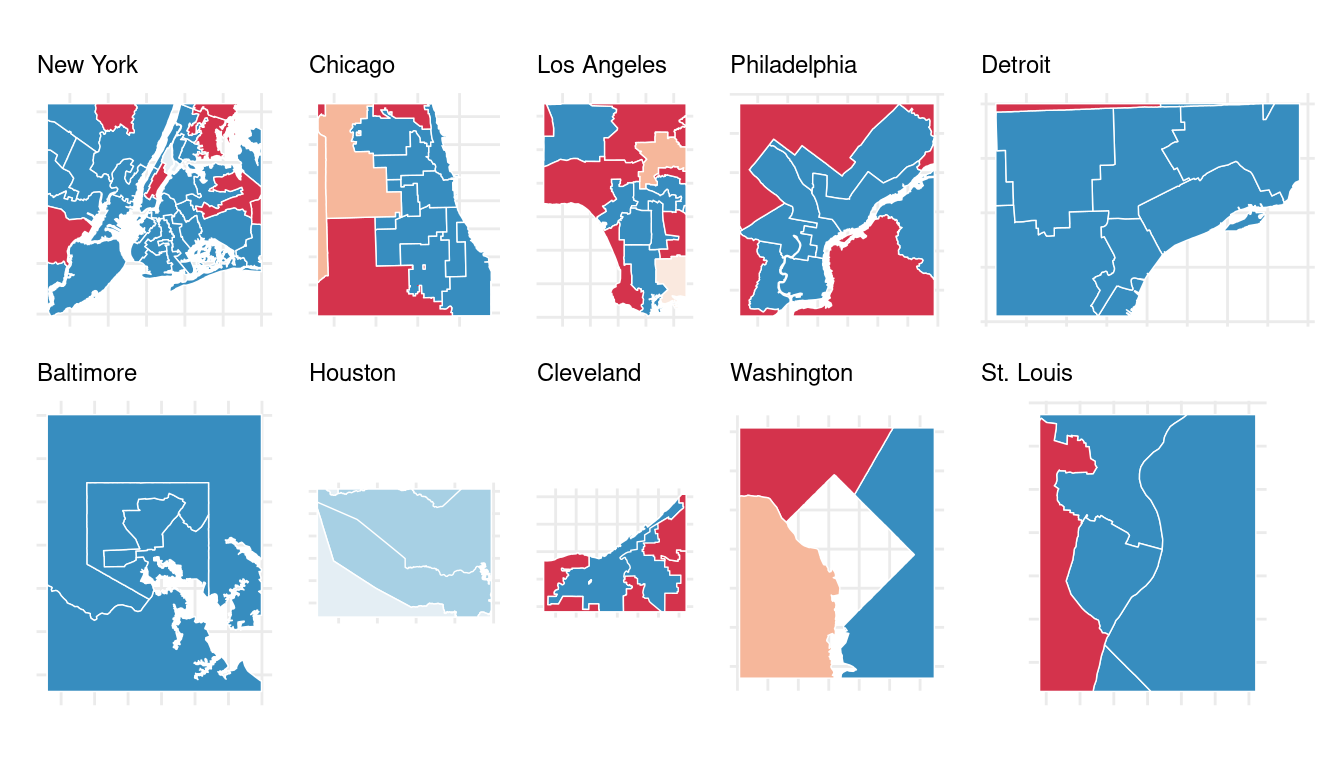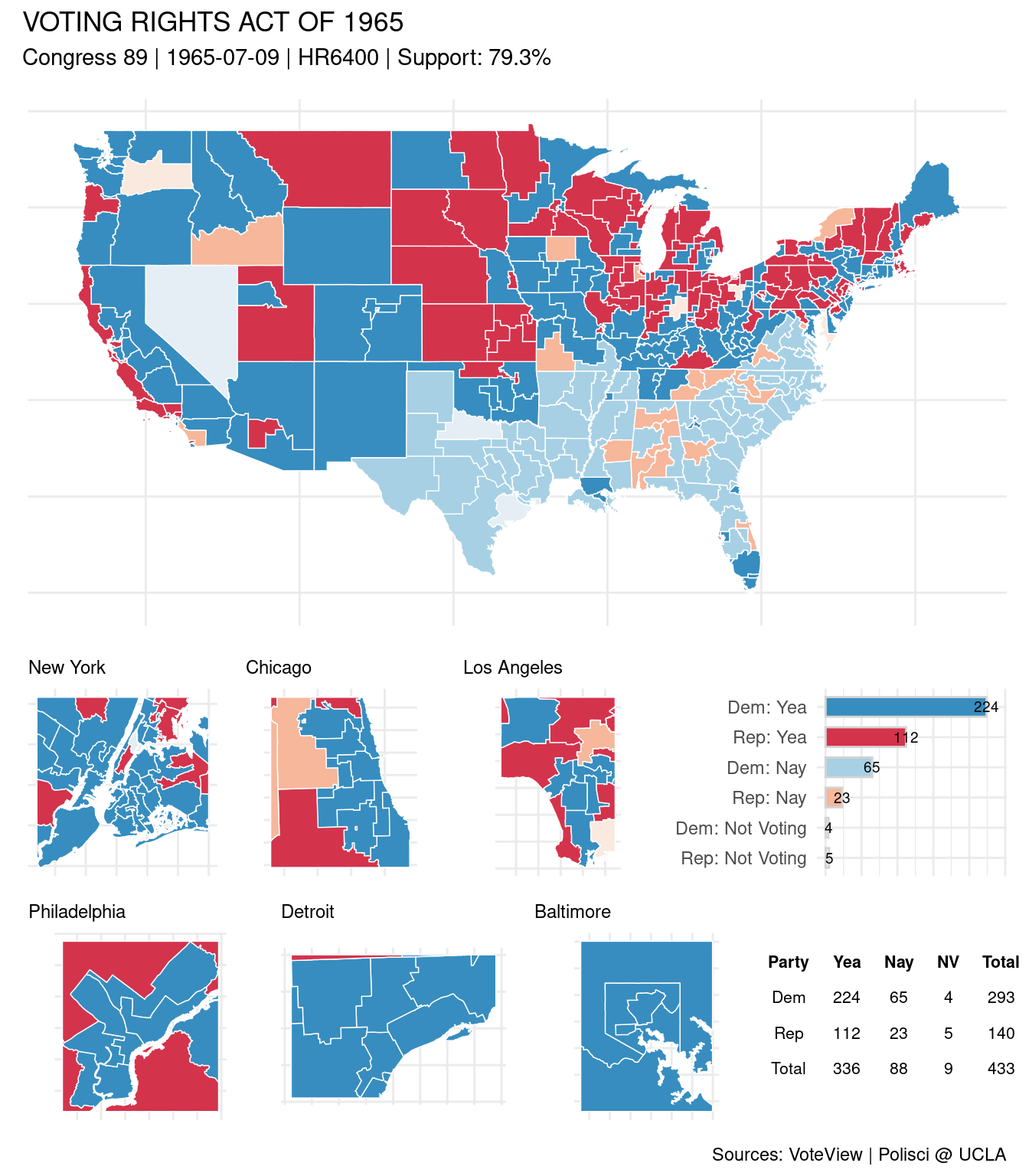# mapping congressional roll calls

## Introduction

A bit of a depot for things-/methods- mapping with R & ggplot, in the context of visualizing historical roll calls from the US House of Representatives. Roll call data accessed via VoteView and the RVoteview package; shapefiles for historical US Congressional Districts downloaded from the Political Science Dept @ UCLA. Visual summary via the patchwork package.

Here we use as examples the Voting Rights Act of 1965 and the Bayh–Celler amendment (circa 1969), a proposed amendment that would have replaced the Electoral College with a system based on the popular vote.

#  devtools::install_github("voteview/Rvoteview")
library(patchwork)
library(tidyverse)
library(tigris); options(tigris_use_cache = TRUE, tigris_class = "sf")
nonx <- c('78', '69', '66', '72', '60', '15', '02')

states <- tigris::states(cb = TRUE) %>%
data.frame() %>%
select(STATEFP, STUSPS) %>%
rename(state_abbrev = STUSPS)

## Historical urban centers

Most populous US cities by decade, from 1790 to 2010; scraped from Wikipedia. For zooming-in on district roll call results for, eg, the ten most populous cities during a given congress.

wiki <- 'https://en.wikipedia.org/wiki/List_of_most_populous_cities_in_the_United_States_by_decade'

decade <- seq(from = 1780, to = 2010, by = 10)
rvest::html_nodes("table") %>%
rvest::html_table(fill = TRUE)

pops <- lapply(2:24, function(x) {
y <- pops_list[[x]] %>%
select(1:4) %>%

colnames(y) <- c('rank', 'city', 'state', 'pop', 'decade')
return(y) }) %>%
bind_rows() %>%
mutate(pop = as.integer(gsub("[^0-9]", "", pop)))

Most populated US cities circa 1800:

1 New York New York 60514 1800
3 Baltimore Maryland 26514 1800
4 Boston Massachusetts 24937 1800
5 Charleston South Carolina 18824 1800
6 Northern Liberties Pennsylvania 10718 1800
7 Southwark Pennsylvania 9621 1800
8 Salem Massachusetts 9457 1800
9 Providence Rhode Island 7614 1800
10 Norfolk Virginia 6926 1800

## Historical congressional districts

Again, via the folks at the Political Science Dept @ UCLA. The Voting Rights Act of 1965 was passed during the 89th congress; a local copy of the shapefile for this congress is loaded below.

fname <- 'districts089'

cd_sf <- sf::st_read(dsn = paste0(cd_directory, fname),
layer = fname,
quiet = TRUE) %>%
mutate(STATEFP = substr(ID, 2, 3),
district_code = as.numeric(substr(ID, 11, 12))) %>%
left_join(states, by = "STATEFP") %>%
filter(!STATEFP %in% nonx) %>%
select(STATEFP, state_abbrev, district_code) 

## VoteView roll call data

Downloading roll call data for a specific bill via RVoteview requires a bit of trial and error; different bill versions and vote types complicate things for the layman.

vra <- Rvoteview::voteview_search('("VOTING RIGHTS ACT OF 1965") AND (congress:89)
AND (chamber:house)') %>%
filter( date == '1965-07-09') %>%
janitor::clean_names()

votes <- Rvoteview::voteview_download(vra$id) names(votes) <- gsub('\\.', '_', names(votes)) A quick re-structure of the roll call output: big_votes <- votes$legis_long_dynamic %>%
left_join(votes$votes_long, by = c("id", "icpsr")) %>% filter(!grepl('POTUS', cqlabel)) %>% group_by(state_abbrev) %>% mutate(n = length(district_code)) %>% ungroup() %>% mutate(avote = case_when(vote %in% c(1:3) ~ 'Yea', vote %in% c(4:6) ~ 'Nay', vote %in% c(7:9) ~ 'Not Voting'), party_code = case_when(party_code == 100 ~ 'Dem', party_code == 200 ~ 'Rep' ), Party_Member_Vote = paste0(party_code, ': ', avote), ## fix at-large -- district_code = ifelse(district_code %in% c(98, 99), 0, district_code), district_code = ifelse(n == 1 & district_code == 1, 0, district_code), district_code = as.integer(district_code)) %>% select(-n) #Members who represent historical “at-large” districts are ##assigned 99, 98, or 1 in various circumstances. Per VoteView. ## Roll call stats big_votes$Party_Member_Vote <- factor(big_votes$Party_Member_Vote) big_votes$Party_Member_Vote <-
factor(big_votes$Party_Member_Vote, levels(big_votes$Party_Member_Vote)[c(3,6,1,4,2,5)])

### /Results

summary <- big_votes %>%
group_by(party_code, avote) %>%
count() %>%
rename(Party = party_code,
NV = Not Voting) %>%
select(Party, Yea, Nay, NV, Total)
Table 1: Roll call results for the VRA
Party Yea Nay NV Total
Dem 224 65 4 293
Rep 112 23 5 140
Total 336 88 9 433

### /By party affiliation

roll <- big_votes %>%
group_by(Party_Member_Vote) %>%
count() %>%
ungroup() %>%
rename(Vote = Party_Member_Vote)

rsum <- roll %>%
ggplot(aes(x=Vote, y=n, fill= Vote, label = n)) +
geom_col(width=.65, color = 'lightgray') +
geom_text(size = 2.5) +
scale_x_discrete(limits = rev(levels(roll$Vote)))+ coord_flip() + ylim (0, 240) + theme_minimal() + theme(axis.title.x=element_blank(), axis.text.x=element_blank(), axis.title.y=element_blank(), #axis.text.y=element_blank(), legend.position = 'none') rsum + ggtitle(vra$short_description)### /By congressional district

cd_sf_w_rolls <- cd_sf %>%

main1 <- cd_sf_w_rolls %>%
ggplot() +
geom_sf(aes(fill = Party_Member_Vote),
color = 'white',
size = .25) +

theme_minimal() +
theme(axis.title.x=element_blank(),
axis.text.x=element_blank(),
axis.title.y=element_blank(),
axis.text.y=element_blank(),
legend.position = 'none') # +

main1 + ggtitle(vra$short_description)## Zooming in to urban centers A zoom function for closer inspection of roll call results in urban areas. The sub_geo parameter is used to specify a vector of city/state pairs (eg, “Chicago, Illinois”) to be geocoded via the tmaptools::geocode_OSM function. The geo parameter specifies the full map – as sf object. maps_get_minis <- function(sub_geos, geo){ lapply(sub_geos, function(x) { lc <- tmaptools::geocode_OSM (q = x, as.sf = T) lc$bbox <- sf::st_set_crs(lc$bbox, sf::st_crs(geo)) cropped <- sf::st_crop(geo, lc$bbox)

ggplot() + geom_sf(data = cropped,
aes(fill = Party_Member_Vote),
color = 'white', size = .25) +

# ggsflabel::geom_sf_text_repel(data = cropped,
#                               aes(label = district_code),
#                               size = 2.2) +

theme_minimal() +
theme(axis.title.x=element_blank(),
axis.text.x=element_blank(),
axis.title.y=element_blank(),
axis.text.y=element_blank(),
plot.title = element_text(size=9),
legend.position = 'none') +

### /Zooms

Roll call results for the VRA (1965) – zoomed in to the ten most populous US cities during the 1960s.

patchwork::wrap_plots(sub_maps, nrow = 2)## A patchwork perspective

t2 <- gridExtra::tableGrob(summary,
rows = NULL,
theme = gridExtra::ttheme_minimal(base_size = 8))

p0 <- sub_maps[] + sub_maps[] + sub_maps[] +
rsum + patchwork::plot_layout(nrow = 1, widths = c(1,1,1,1))

p1 <- sub_maps[] + sub_maps[] + sub_maps[] +
t2 + patchwork::plot_layout(nrow = 1, widths = c(1,1,1,1))

p2 <- p0/p1 + patchwork::plot_layout(nrow = 2)#, heights = c(4, 1))
main1 / p2  + patchwork::plot_layout(ncol = 1, heights = c(5, 4)) +
plot_annotation(
title = vra$short_description, subtitle = paste0('Congress ', vra$congress, ' | ',
vra$date, ' | ', vra$bill_number, ' | ',
'Support: ', round(vra\$support, 1), '%'),
caption = 'Sources: VoteView | Polisci @ UCLA')